WHCSRL 技术网

# 【JavaSE】 - 运算符

## 🐱‍🏍系列文章目录1. 算数运算符
2. 逻辑运算符
3. 位运算符
4. 条件运算符

## 🦝算数运算符

1. 基本算数运算符
2. 增量算数运算符（复合运算符）
3. 自增/自减运算符

## 🤢基本算数运算符

'+'运算符

``````public class Operator {

public static void main(String[] args) {

int a = 10;
int b = 20;
System.out.println(a+b);

}

}
```1234567891011```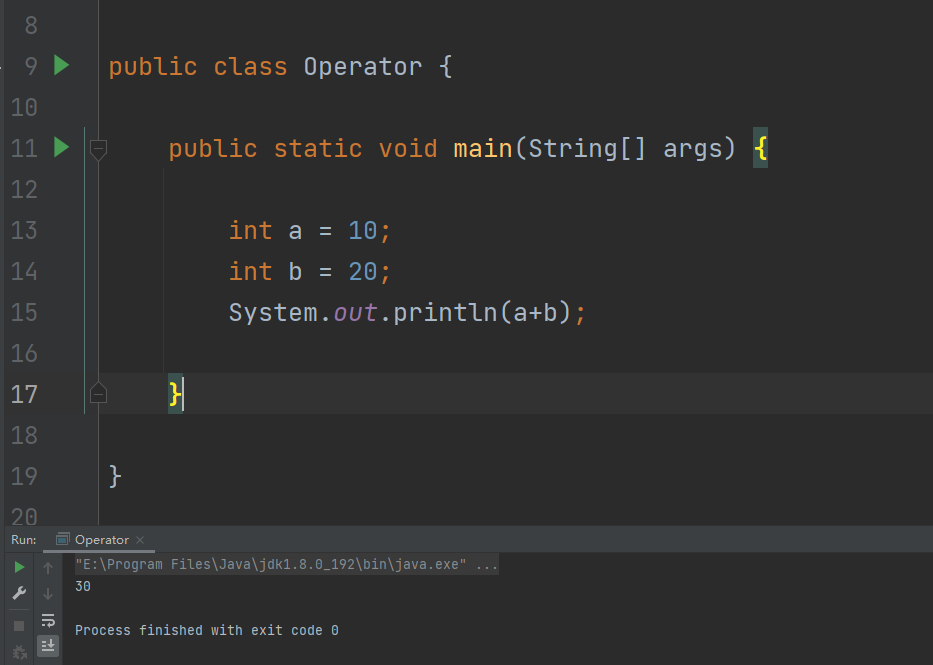'-'运算符

``````public class Operator {

public static void main(String[] args) {

int a = 10;
int b = 5;
System.out.println(a - b);

}

}
```1234567891011```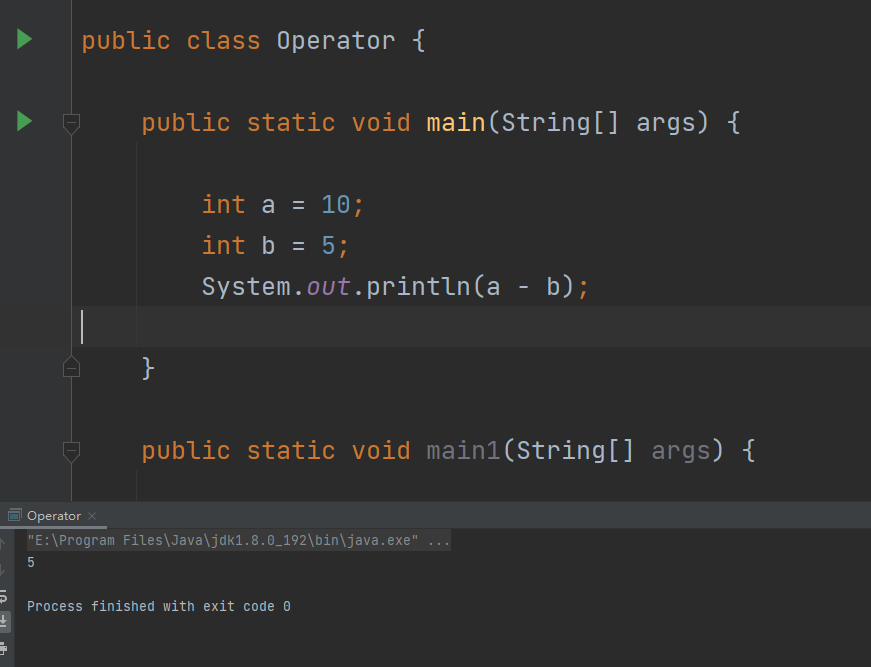'*'运算符

``````public class Operator {

public static void main(String[] args) {

int a = 10;
int b = 5;
System.out.println(a*b);

}

}
```1234567891011```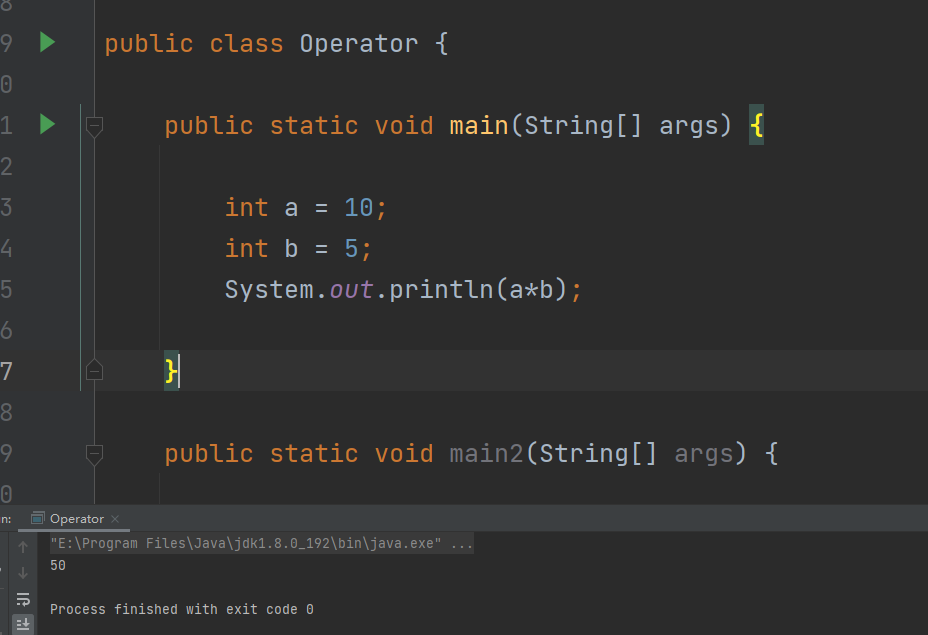'/'运算符

1. 除数不能为0。

``````public class Operator {

public static void main(String[] args) {

int a = 10;
int b = 0;
System.out.println(a/b);

}

}
```1234567891011```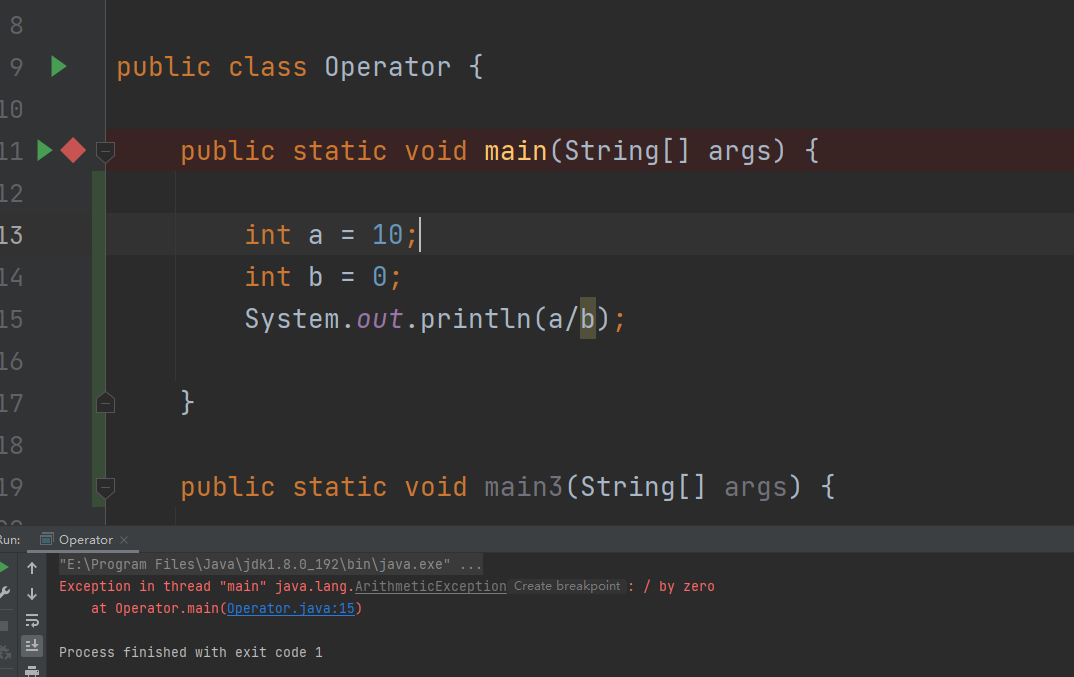1. 整型 / 整型的结果只能是整型。

``````public class Operator {

public static void main(String[] args) {

int a = 10;
int b = 3;
double c = a / b;
System.out.println(c);

}

}
```123456789101112```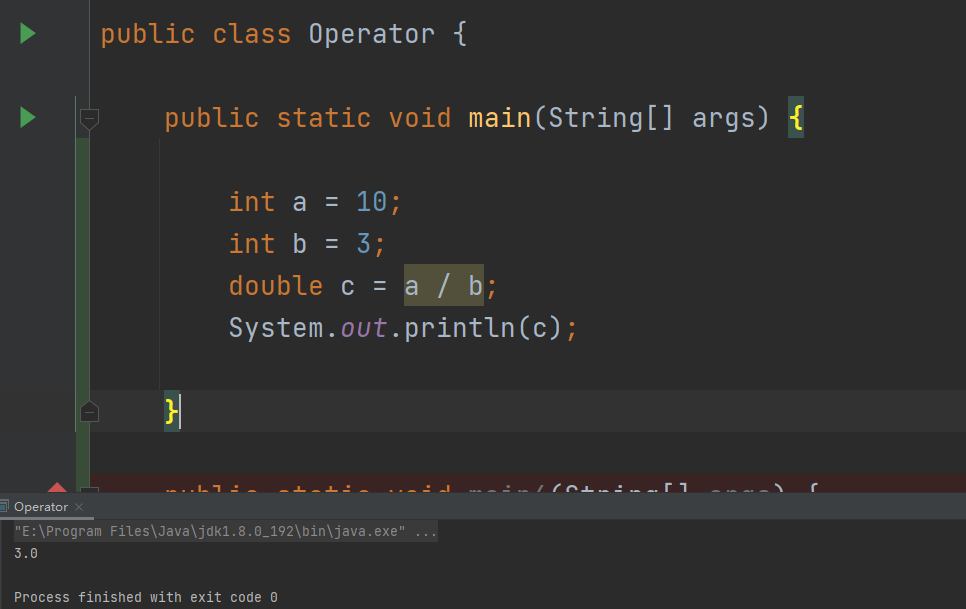'%%%%%%%%%%%%%%%%'运算符

1. 浮点数取模运算。

• 浮点数 %%%%%%%%%%%%%%%% 浮点数，
• 整数 %%%%%%%%%%%%%%%% 浮点数
• 浮点数 %%%%%%%%%%%%%%%% 整数

``````public class Operator {

public static void main(String[] args) {

double a = 12.5;
double b = 3.5;
System.out.println(a%%%%%%%%%%%%%%%%b);

int x = 10;
double y = 3.5;
System.out.println(x%%%%%%%%%%%%%%%%y);

double m = 10.5;
int n = 4;
System.out.println(m%%%%%%%%%%%%%%%%n);

}

}
```12345678910111213141516171819```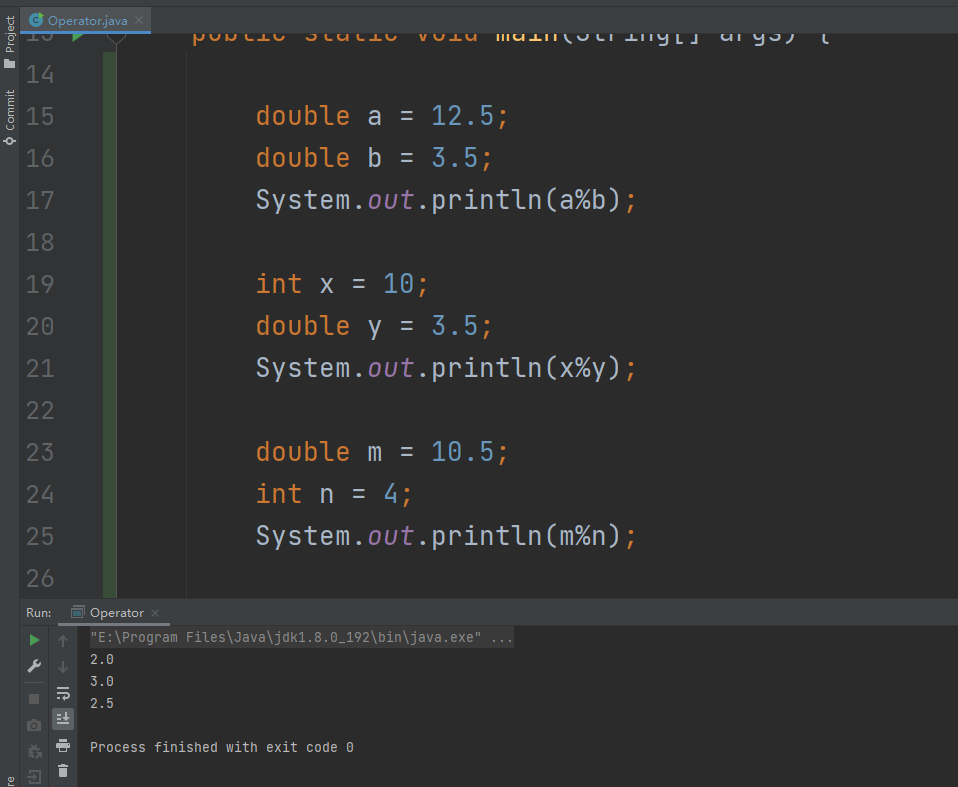1. 负数取模运算

``````public class Operator {

public static void main(String[] args) {

int a = 10;
int b = -3;
System.out.println(a%%%%%%%%%%%%%%%%b);

}

}
```1234567891011```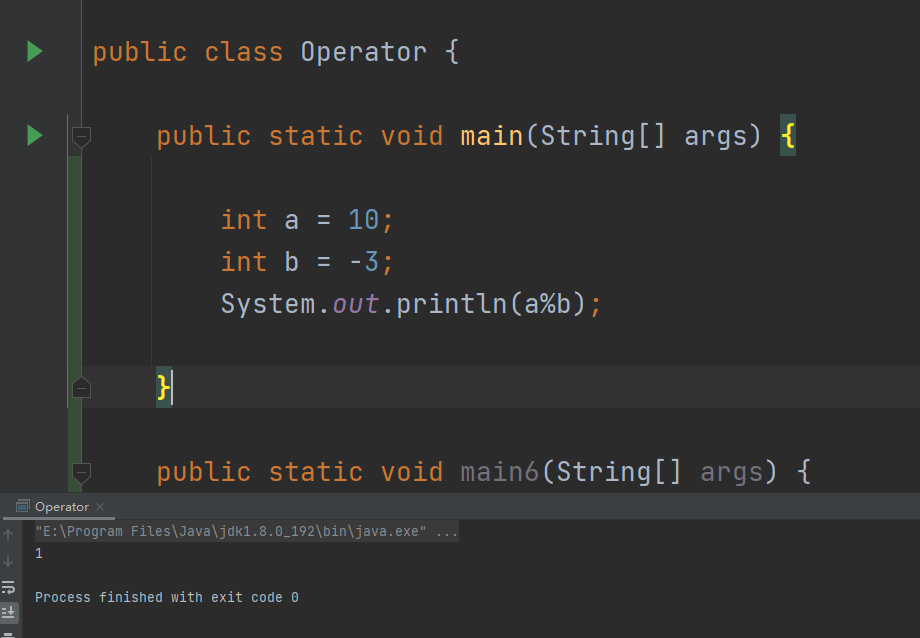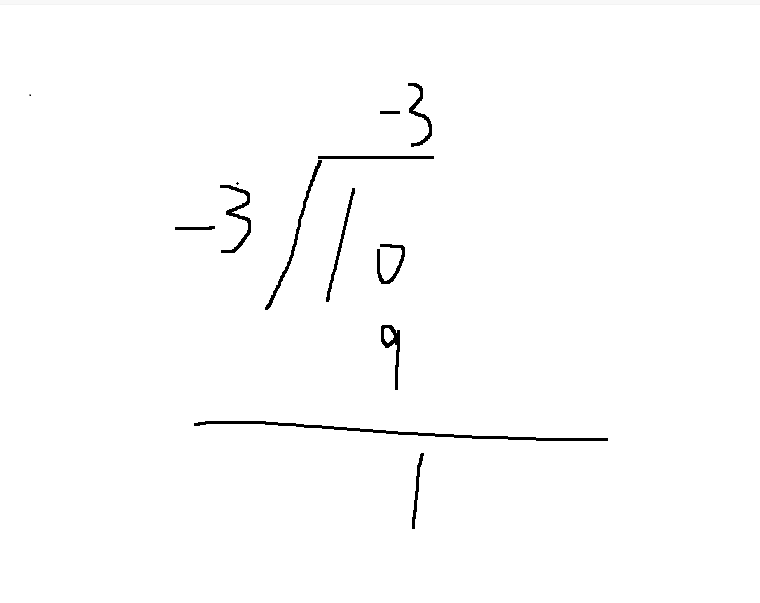## 🍚增量算数运算符

+=，-=，*=，/=。%%%%%%%%%%%%%%%%=，>>=等等

``````a += 1;
```1```

``````a = a + 1;
```1```

Java基础语法 - Java数据类型

``````public class Operator {

public static void main(String[] args) {

int a = 10;
a = a + 2.5;
System.out.println(a);

}

}
```1234567891011```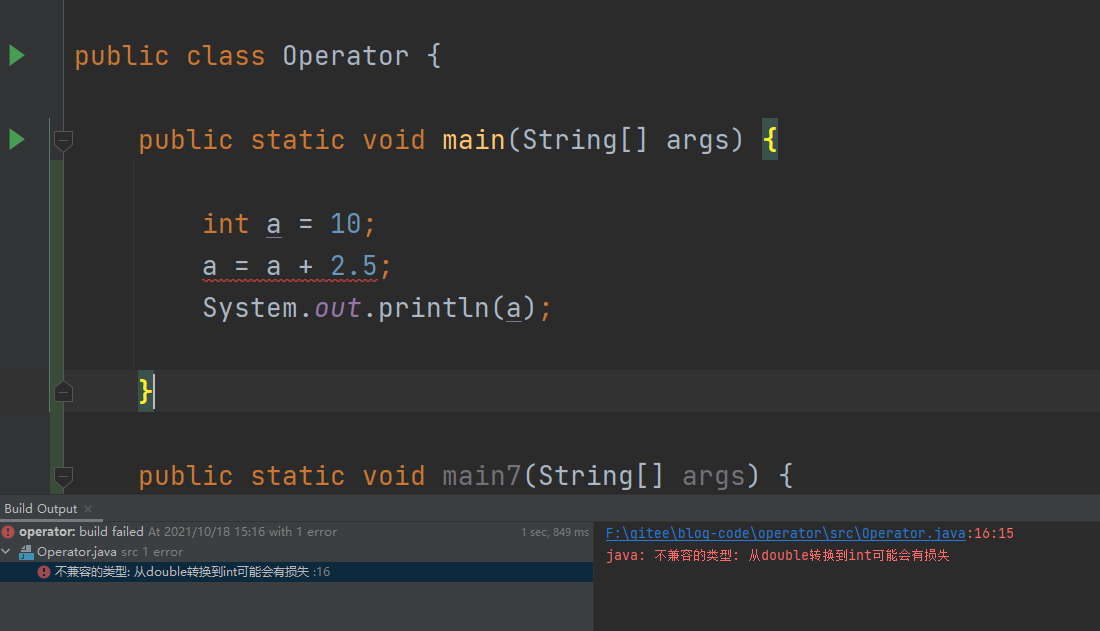``````public class Operator {

public static void main(String[] args) {

int a = 10;
a += 2.5;
System.out.println(a);

}

}
```1234567891011```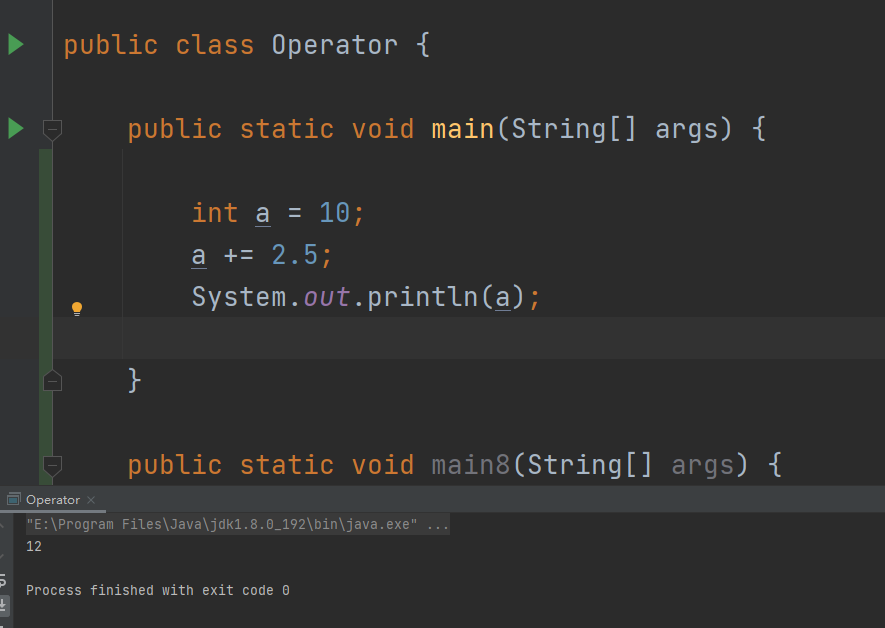## 🔨自增/自减运算符

``````public class Operator {

public static void main(String[] args) {

int a = 10;
int b = 0;
int c = 0;
b = ++a;
c = a++;
System.out.println(b);
System.out.println(c);
System.out.println(a);

}

}
```12345678910111213141516```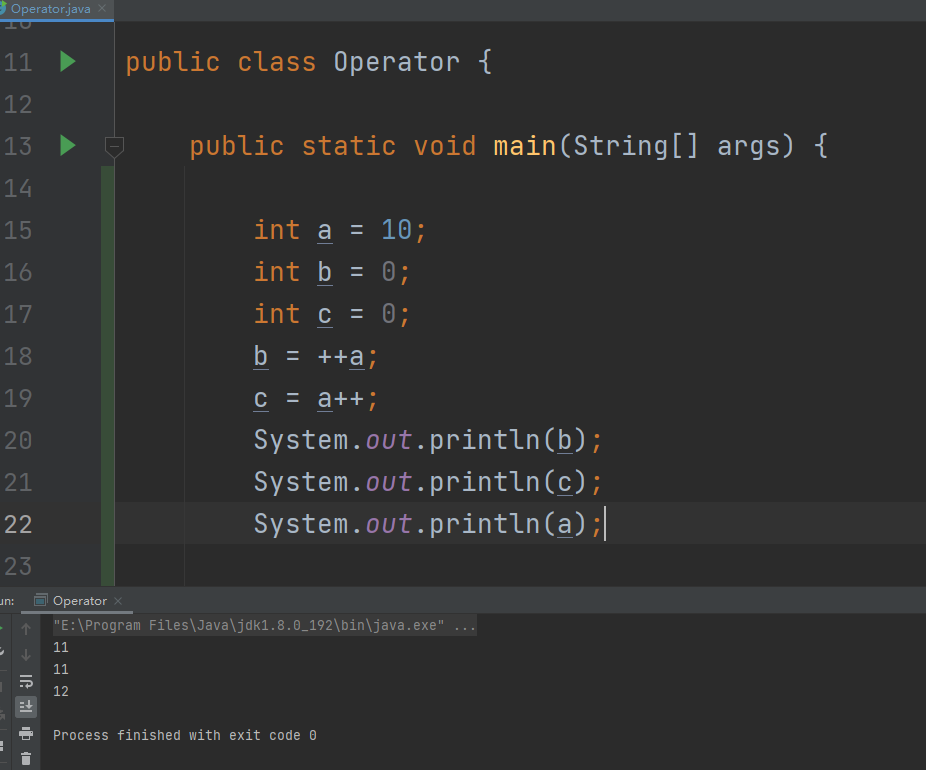``````b = ++a;
```1```

a先自增1后再赋值给b，所以b等于11。

``````c = a++;
```1```

1. 基本逻辑运算符
2. 关系操作运算符

## 🥪基本逻辑运算符

1. 与运算（&&）
2. 或运算（||）
3. 非运算（！）

1. 与运算：

1. 或运算：

1. 非运算

``````public class Operator {

public static void main(String[] args) {

boolean a = true;
boolean b = false;

System.out.println(a && b);
System.out.println(a || b);
System.out.println(!a);
System.out.println(!b);

}

}
```123456789101112131415```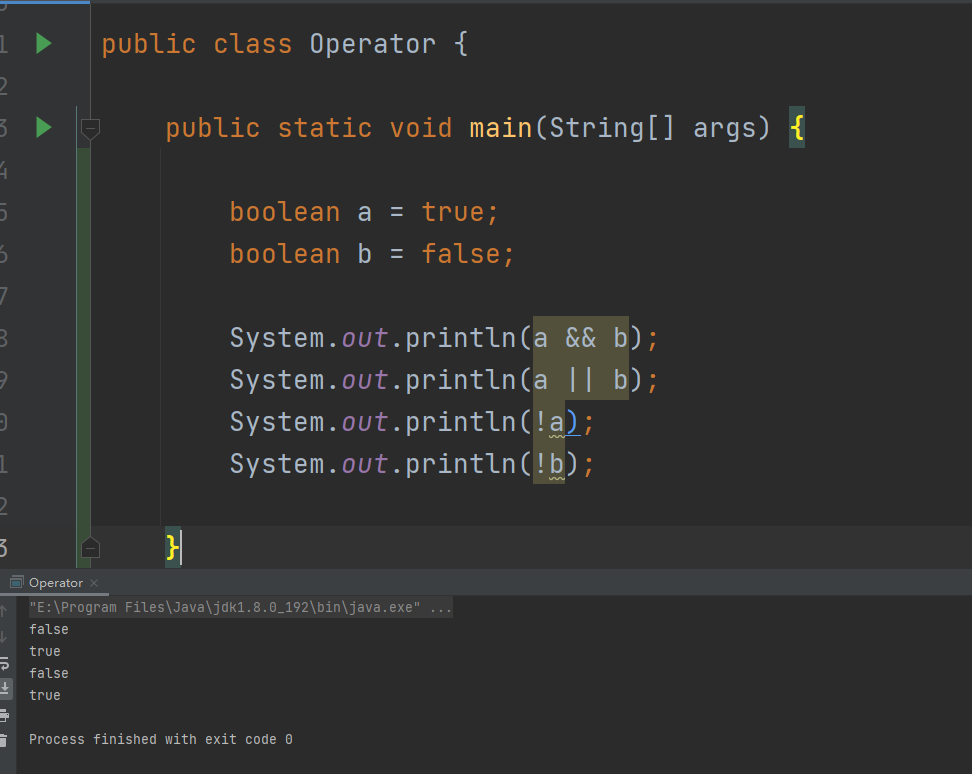### 🤳短路运算

1. 对于逻辑与运算（&&）：如果左侧表达式结果为false，则不会进行右侧表达式的运算。
2. 对于逻辑或运算（||）：如果左侧表达式的结果为true，则不会进行对右侧表达式的运算。

``````public class Operator {

public static void main(String[] args) {

System.out.println(10 > 20 && 0 == 10 / 0);
System.out.println(10 < 20 || 0 == 10 / 0);

}

}
```12345678910```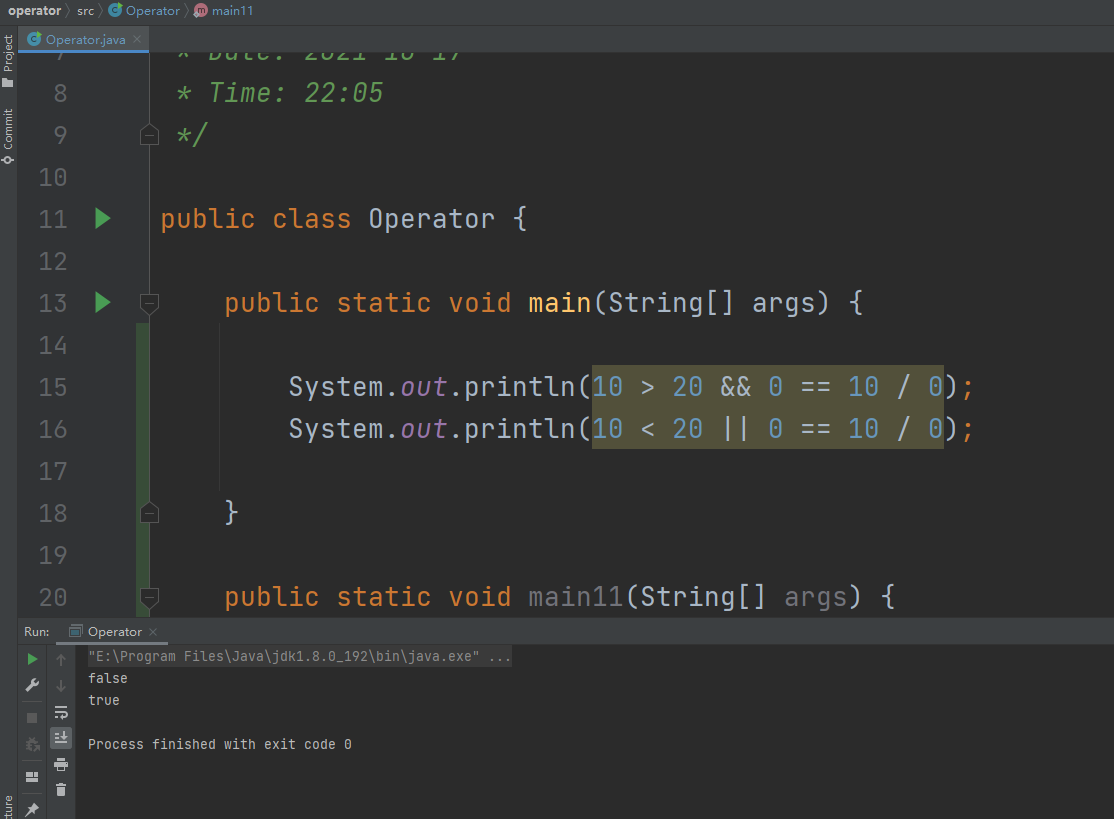## 🚆关系操作运算符

==，！=，>=，<=，<，>

``````public class Operator {

public static void main(String[] args) {

int a = 10;
int b = 20;

System.out.println(a == b);
System.out.println(a != b);
System.out.println(a > b);
System.out.println(a < b);
System.out.println(a >= b);
System.out.println(a <= b);

}

}
```1234567891011121314151617```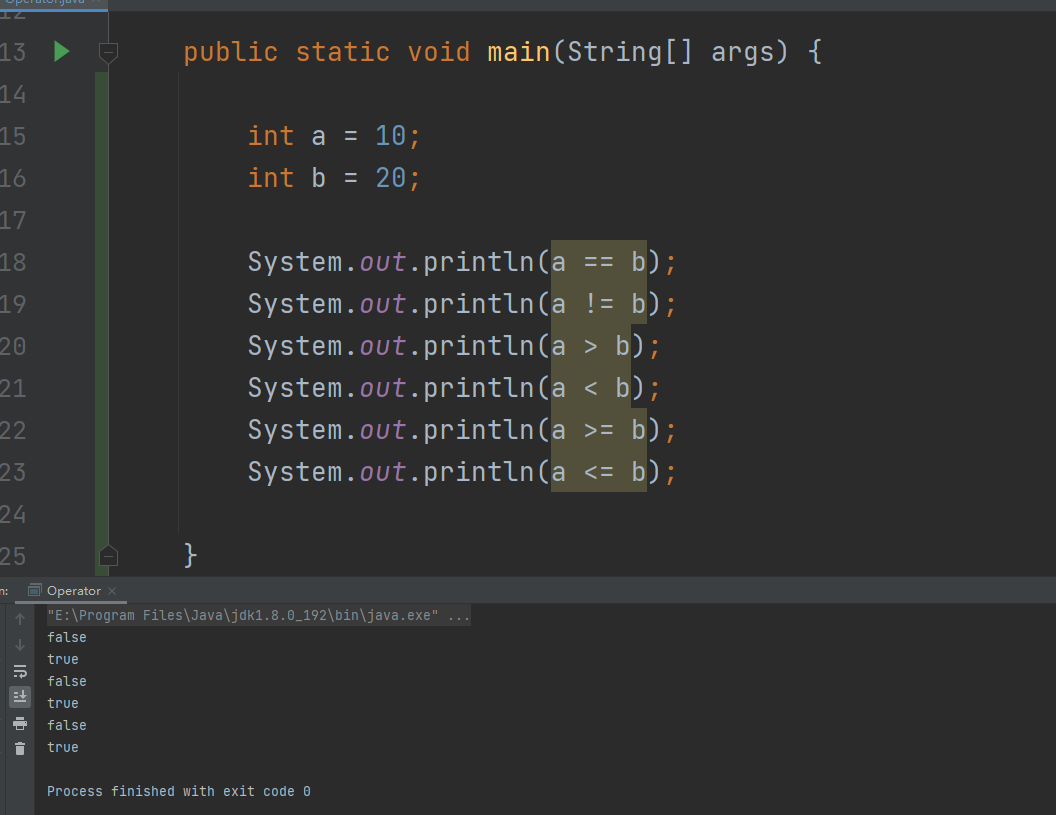1. 按位运算操作符
2. 移位运算操作符

## 🚲按位运算操作符

Java中的按位运算操作符有：

1. 按位与（&）操作符

``````10010101001010011010010110101010
00010110101011101111010110001010 -- &
00010100001010001010010110001010
```123```

1. 按位或（|）操作符

``````01000010011101110100101000111010
10110011010010111100001010000100 -- |
11110011011111111100101010111110
```123```

1. 按位异或（^）操作符``````10010110110001011010010110100111
00101011010010011010100111011101 - ^
01000010011100111111001110000101
```123```

## 🌹移位运算操作符

1. 左移操作符（<<）
2. 右移操作符（>>）
3. 无符号右移操作符（>>>）

``````10000000000000000000000000000011 - -3原码
11111111111111111111111111111100 - 反码
11111111111111111111111111111101 - 补码

11111111111111111111111111111101 - -3
11111111111111111111111111111010  -  左移1位 - 补码
10000000000000000000000000000101
10000000000000000000000000000110 - 原码 - -6

11111111111111111111111111111101 - -3
11111111111111111111111111111110  -  右移1位 - 补码
10000000000000000000000000000001
10000000000000000000000000000010 - 原码 - -2

11111111111111111111111111111101 - -3
01111111111111111111111111111110  -  无符号右移1位 - 正数
```12345678910111213141516```

``````public class Operator {

public static void main(String[] args) {

int a = -3;

System.out.println(a << 1);
System.out.println(a >> 1);
System.out.println(a >>> 1);

}

}
```12345678910111213```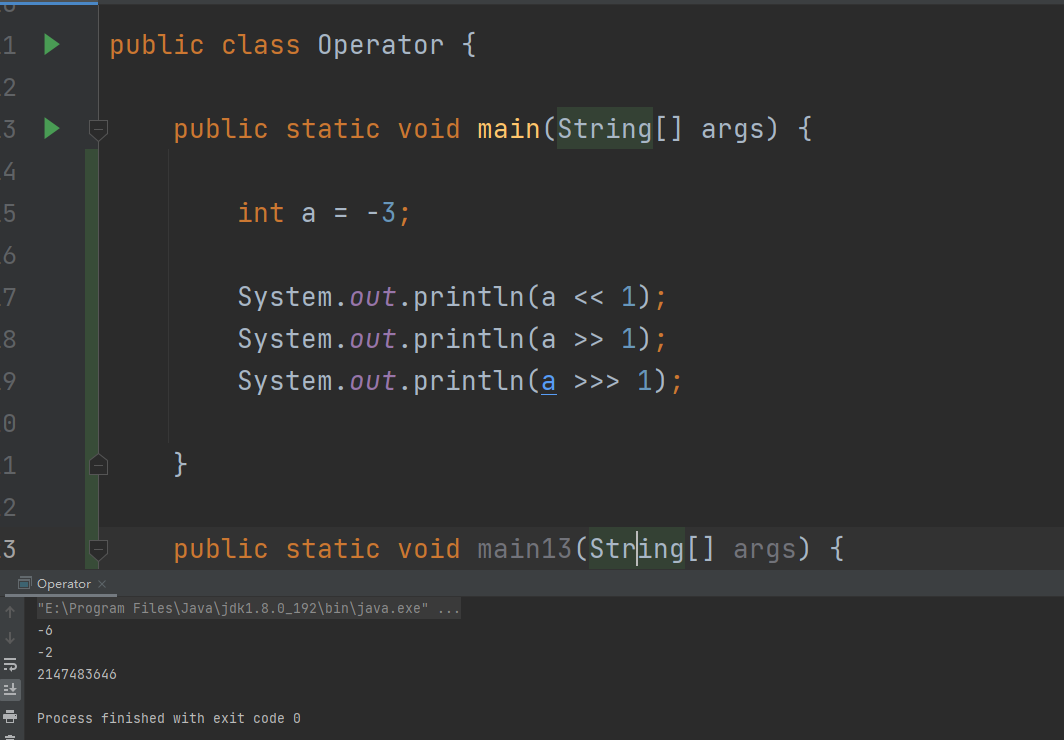1. 左移1位，相当于原来的数乘以2
2. 右移1位，相当于原来的数除以2

## 🐱‍🚀条件操作符（三目操作符）

``````public class Operator {

public static void main(String[] args) {

int a = 10;
int b = 20;
int max = a > b ? a : b;
System.out.println(max);

}

}
```123456789101112```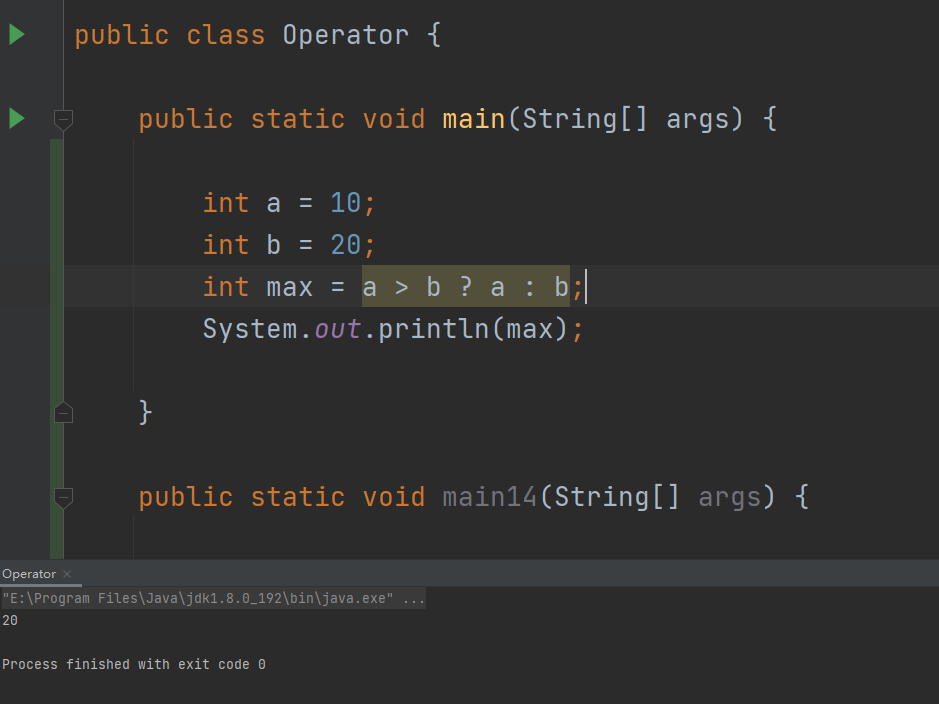## 🍎注释

Java中的代码注释分为以下三种：

1. 单行注释：//（注释内容）
2. 块注释（多行注释）：/ *（注释内容） * /
3. 文档注释：/**（注释内容）*/

1. 内容准确：要求注释内容应该和代码相符，如果修改了代码应该及时更新注释。
2. 篇幅精炼：注释不适宜长篇大论，能让自己和别人看懂即可。
3. 使用中文：代码的注释是给自己和同事看的，不要炫耀自己的英文水平，应该最大可能的让自己和别人看懂。
4. 积极向上：不得出现辱骂国家，有损国家形象或者辱骂领导的语句词汇，不要涉及政治敏感词汇

## 🍃Java关键字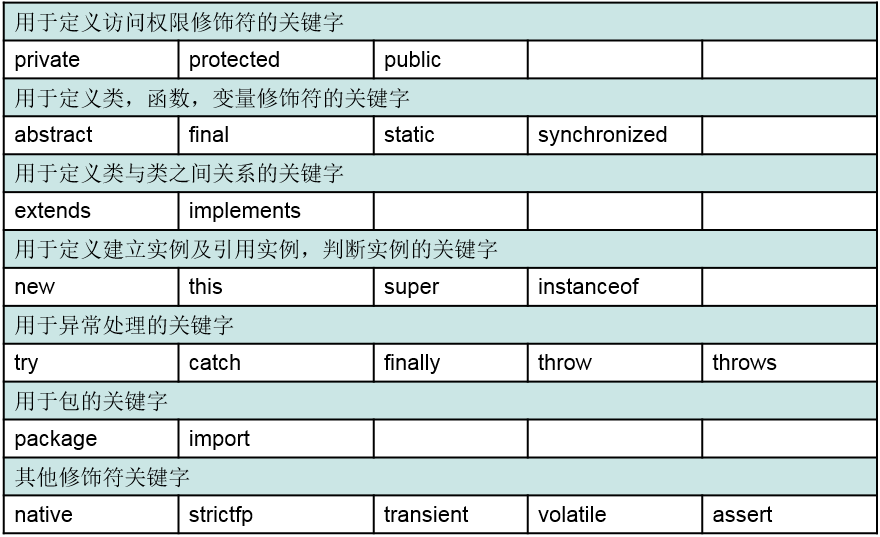## 😁总结

👍点赞👍 + 👀关注👀 + ✔️收藏✔️